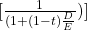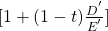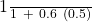Cost of Capital | IFT World
IFT Notes for Level I CFA® Program
IFT Notes for Level I CFA® Program

# Part 3

## 4. Estimating Beta

A firm’s beta is used to estimate its required return on equity. Beta is a measure of the company’s systematic or market related risk. Riskier firms will have higher betas, whereas less risker firms will have lower betas.

### Estimating Beta for Public Companies

For a public company, beta is computed by regressing the return on the stock with the return on the market. The regression equation is: Ri= αi+ βi*RM. The return on market is plotted on the x-axis as an independent variable. The return on stock is plotted on the y-axis as a dependent variable. The slope of the best-fit line through this data is the regression beta.

Regression beta is also called an unadjusted or raw historical beta. Such a beta estimate is influenced by:

• The choice of index used to represent the market. For US equities, indexes such as the S&P 500 and NYSE are used.
• The length of data and frequency of observation. Often, five years of monthly data are used.

Adjusted beta: In the long-term, beta has been observed to have a mean-reverting value of 1. Beta for high-risk stocks is more than 1 but it gradually drifts towards 1. Similarly, beta for low-risk stocks is less than 1 but it gradually drifts towards 1. Therefore, some analysts adjust the raw beta using the ‘Blume method’ as shown below:

Example: If the beta from a regression of an asset’s returns on the market return is 1.20, calculate its adjusted beta.

Solution:

Adjusted beta = (2/3)(1.20) + (1/3)(1.0) = 1.13.

### Estimating Beta for Thinly Traded and Nonpublic Companies

For companies that are thinly traded or private companies, beta cannot be determined through regression, as market price information is not readily available. So, betas of publicly traded comparable companies are used as a proxy. A four-step process is followed to make adjustments:

Step 1: Select the benchmark public company

Step 2: Estimate benchmark’s beta

Step 3: Unlever the benchmark’s beta, i.e., estimate the beta without the impact of debt (or leverage). The formula for unlevering beta is:

βU = βEwhere:

βU = unlevered beta

D = debt of benchmark company

E = equity of benchmark company

βE = beta of benchmark company

Step 4: Lever the beta to reflect the subject company’s financial leverage. The formula for calculating the beta of the subject company is:

β’E = βUwhere:

β’E = beta of subject company

D’ = debt of subject company

E’ = equity of subject company

βU = unlevered beta (benchmark)

Example

AA Corp. is a large conglomerate and wants to determine the equity beta of its food division. This division has a D/E ratio of 0.7. The tax rate is 40%. A comparable publicly traded food company has an equity beta of 1.2 and a D/E ratio of 0.5. What is the equity beta of AA’s food division?

Solution:

We can calculate the equity beta of AA’s food division as:

1. Unlevered beta of publicly traded food company = 1.2 *= 0.923
2. Levered equity beta of AA’s food division = 0.923 [1 + 0.6 x 0.7] = 1.31

Inference: Since AA’s food division has more debt than the publicly traded company, it is riskier and has a higher beta value.

LIVE WEEKEND CLASSES *For 2024 Exam candidates*
This is default text for notification bar